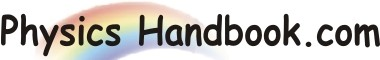HOME TOPICS DEFINITIONS TABLES LAWS INVENTIONS EXPERIMENTS QUIZ VIDEOS
 A B C D E F G H I J K L M N O P Q R S T U V W X Y Z
Nuclear Forces
The strong forces of attraction which firmly hold the nucleons in the nucleus are known as nuclear forces.
The forces holding the nucleus together must be extremely strong. This is clear from the fact that the positively charged protons remain confined to the small volume of the nucleus.
If the nuclear forces are weak, the electrostatic repulsion of the positively charged protons would tend to break the nucleus apart or at the very least, the nucleus would occupy a much larger volume.
Much is not known about the nuclear forces. However it is undoubtedly established that these forces exist between the nucleons i.e. between a neutron and a proton, between two protons and between two neutrons. The stability of the nucleus is accounted for due to the presence of these forces.
Some important properties of nuclear forces are :
They are attractive in character :
They exert attractive forces on the nucleons. Hence they are also called cohesive forces.
They are charge independent :
They are the same between p and n or between p and p or between n and n. These forces do not depend upon the charge on the nucleons.
They are short range forces :
It has been found that they are quite strong for an internucleon distance of the order of 10-15 m but become zero at an internucleon distance of 10 -14 m. Nuclear forces change with distance between the nucleons.
They have saturation character :
They abruptly become zero as the inter-nucleon distance is increased to a certain value. Therefore, nuclear forces do not increase with the increase in the number of nucleons.
They are extremely strong :
Their magnitude is so high that a huge energy is required to divide a nucleus into its constituents. The relative strengths of gravitational (FG), electrostatic (Fe) and nuclear (FB) forces acting in the nucleus is given below,
FG : Fe : FB = 1 : 1036 : 1038
The nuclear forces are dependent on the spin of the nuclei.
Nuclear forces are non-central forces :
The force between two nucleons does not act along the line joining their centers. This shows that nucleons in the nucleus is not spherically symmetric.